# 8 2 Equations Involving Absolute Value 1 Solve

• Slides: 12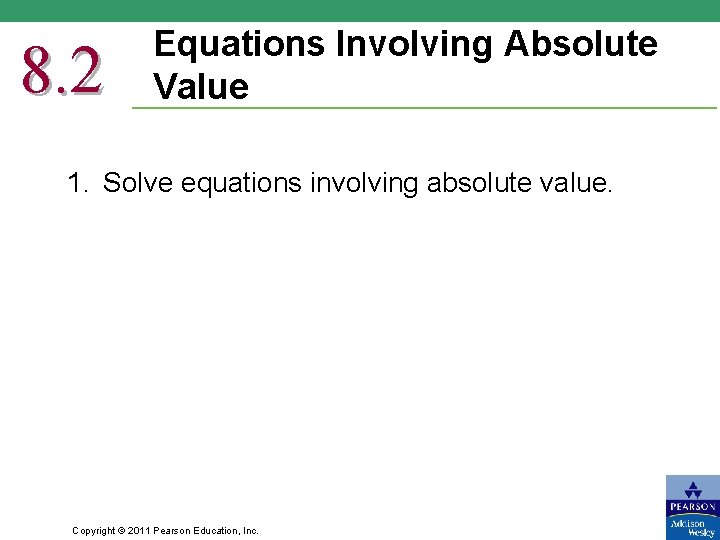8. 2 Equations Involving Absolute Value 1. Solve equations involving absolute value. Copyright © 2011 Pearson Education, Inc.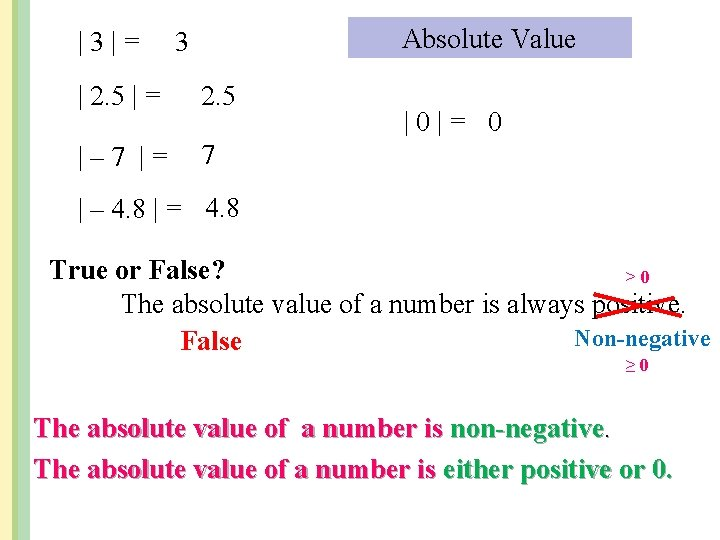|3|= Absolute Value 3 | 2. 5 | = 2. 5 |– 7 |= 7 |0|= 0 | – 4. 8 | = 4. 8 True or False? >0 The absolute value of a number is always positive. Non-negative False ≥ 0 The absolute value of a number is non-negative. The absolute value of a number is either positive or 0.Absolute Value Equations |x|=5 Same x=5 Opposite x=– 5 | x | = – 2 No Solution Two Solutions Absolute Value Property If |x| = a, where x is a variable or an expression and a 0, then x = a or x = a.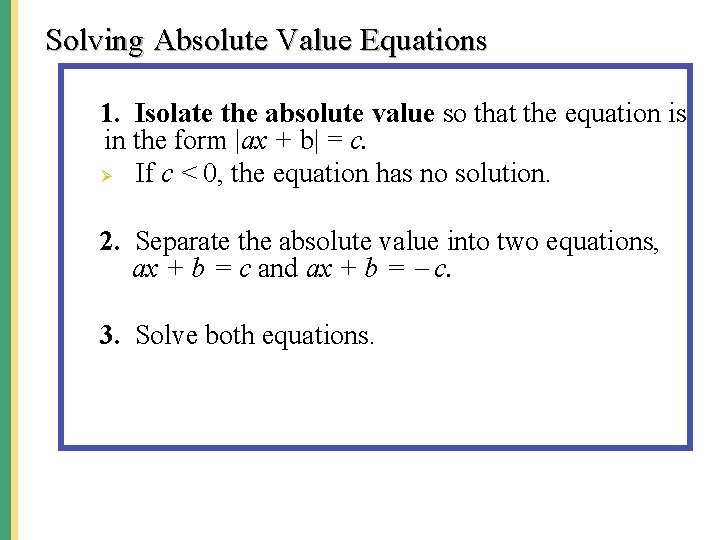Solving Absolute Value Equations 1. Isolate the absolute value so that the equation is in the form |ax + b| = c. Ø If c < 0, the equation has no solution. 2. Separate the absolute value into two equations, ax + b = c and ax + b = c. 3. Solve both equations.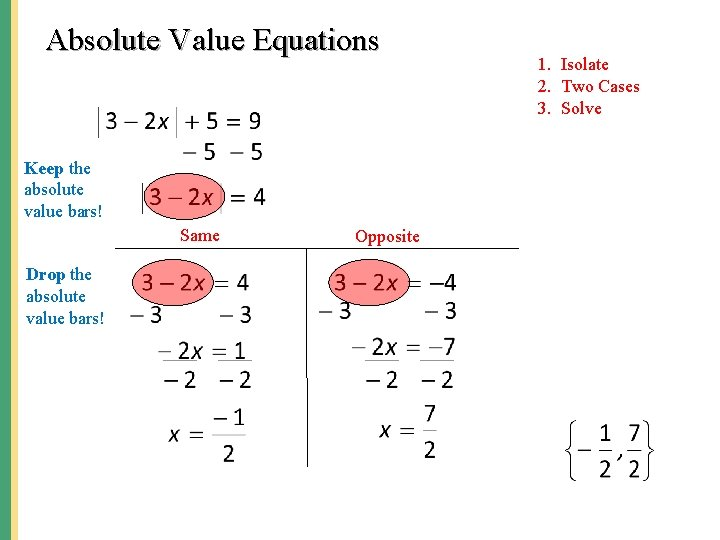Absolute Value Equations Keep the absolute value bars! Same Drop the absolute value bars! Opposite 1. Isolate 2. Two Cases 3. Solve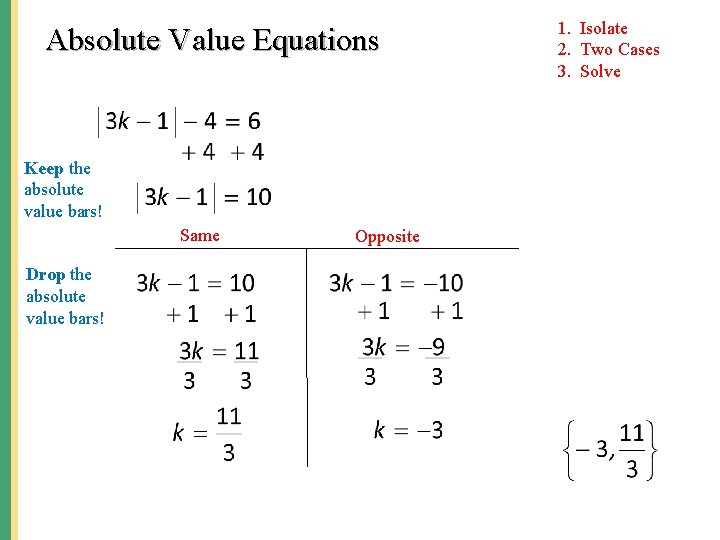Absolute Value Equations Keep the absolute value bars! Same Drop the absolute value bars! Opposite 1. Isolate 2. Two Cases 3. SolveAbsolute Value Equations No Solution 1. Isolate 2. Two Cases 3. Solve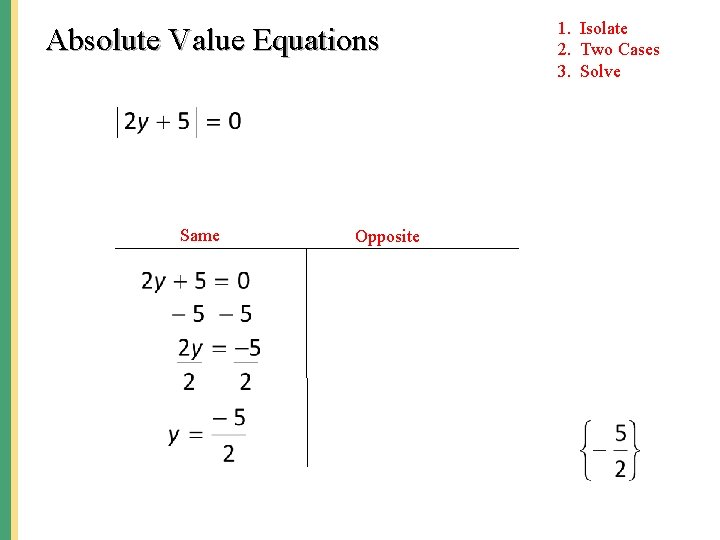Absolute Value Equations Same Opposite 1. Isolate 2. Two Cases 3. Solve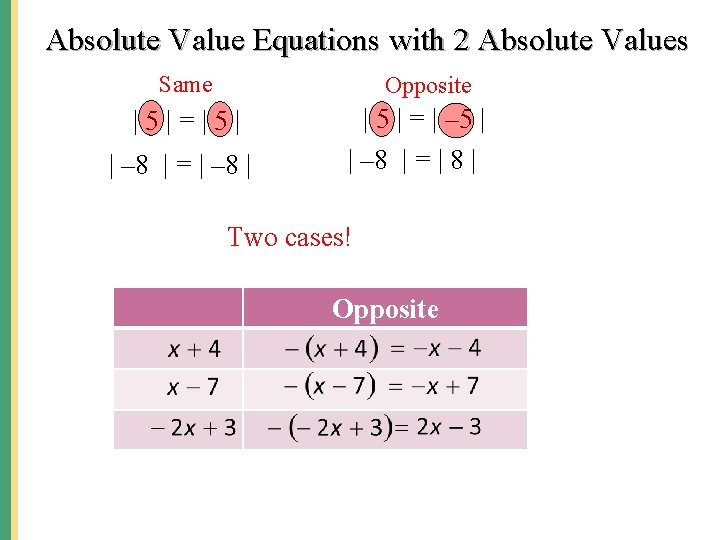Absolute Value Equations with 2 Absolute Values Same Opposite |5|=|5| | – 8 | = | – 8 | | 5 | = | – 5 | | – 8 | = | 8 | Two cases! Opposite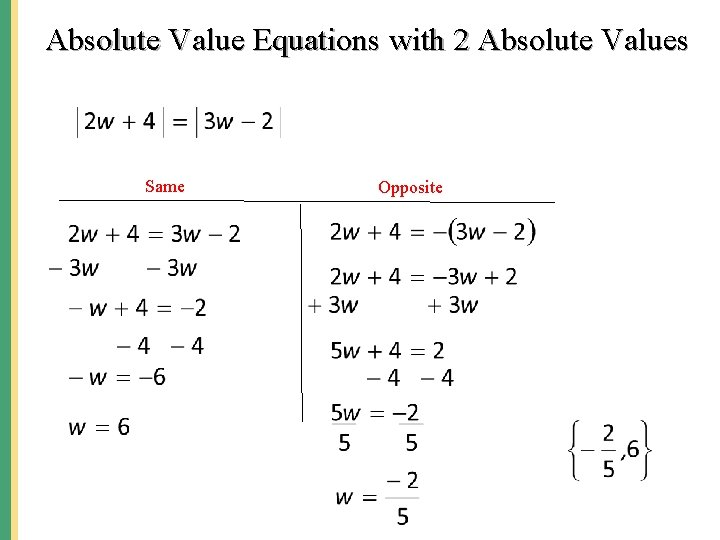Absolute Value Equations with 2 Absolute Values Same OppositeAbsolute Value Equations with 2 Absolute Values Same Opposite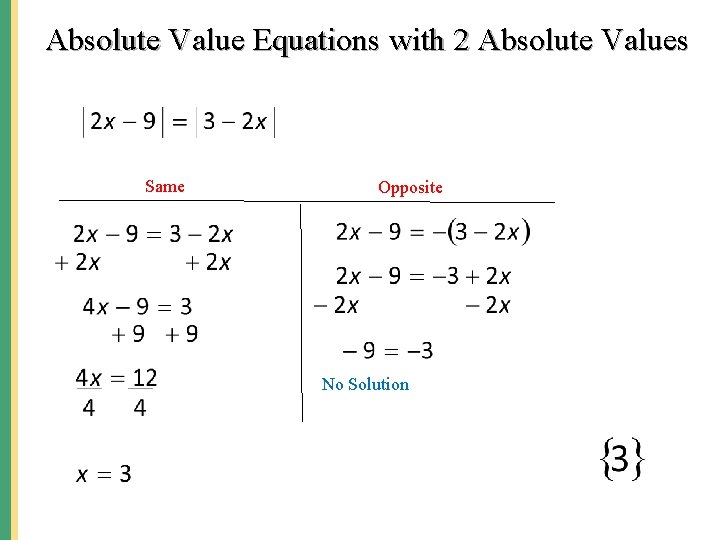Absolute Value Equations with 2 Absolute Values Same Opposite No Solution